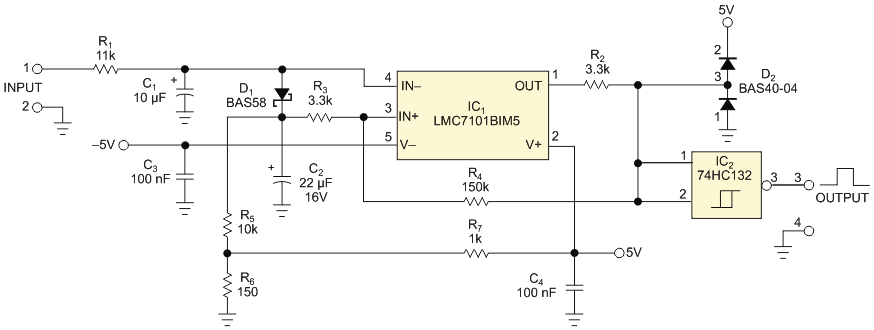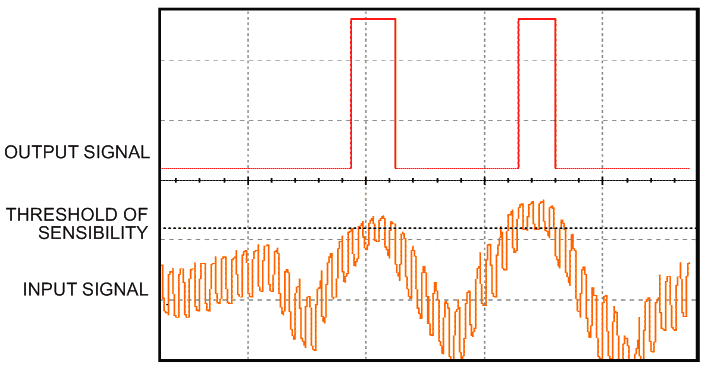# Circuit detects rapidly falling signals and rejects noise

EDN

Detecting a rapidly falling signal over some threshold is important for ultrasonic or location equipment as well as for seismology systems. You can combine a rail-to-rail operational amplifier with a Schmitt-trigger logic gate to perform this function (Figure 1). This example works well in an ultrasound machine. It controls a sample-and-hold amplifier that sets the gain of an AGC (automatic-gain-control) system.Figure 1. This circuit detects signal excursions higher than a set threshold and rejects noise and recognizes fast falling signals.

The circuit works only with positive signals, so the signal must pass through a full-wave rectifier before it is applied to the circuit input. You configure the main part of the circuit, op amp IC1, as a comparator with hysteresis. It produces a high-level output when an input signal is higher than the specified threshold. The output goes to a low level when the input signal begins to fall but only when the input falls faster than an established rate of change or if the level of the input signal will be lower than the established threshold of sensitivity. This circuit detects the moment when a signal is above the established threshold and the falling signal – or a mix of the signal and noise – has higher-than-specified speed.

R1 and C1 form an input lowpass filter to smooth the input signal. You set the values of R1 and C1 to create a filter roll-off for the input signal you are processing. Resistors R3 and R4 establish a small hysteresis, which is necessary so that slow signals with noise don’t cause the output to change state. You set the threshold level with voltage divider R6 and R7. D1, R5, and C2 form a peak detector. R5 establishes a time constant of the discharge of C2 and provides sensitivity to a falling signal’s rate. You establish the circuit’s sensitivity to a falling signal’s rate of change using the time constant, which the values of C2 and R5 set. Hysteresis resistor R4 is more than a decade larger than R5, so the effect of resistors R3 and R4 is negligible.Figure 2. The circuit recognizes a pulse when it falls; noise is exaggerated for clarity.

A rising input signal greater than the threshold charges C2 to approximately the level of the input signal. The output amplifier is at a high level because the voltage on C2 is always lower than the value of the rising input signal due to D1’s voltage drop. When the input drops faster than C2 can discharge through R5, the output level of the device changes to a low level because the voltage on C2 is higher than the value of the falling input signal. If the input signal falls more slowly than the discharge of C2 through resistor R5, the output remains high. Schottky diode D1 prevents the discharge of C2 through the input. R2 and D2 clamp the amplifier’s output to positive values. Feed the clamped signal to Schmitt-trigger logic gate IC2 to give a logic-level output with fast transitions (Figure 2).

EDN